# Psych 216A Lecture 6: Model Reliability¶

Previous lectures have covered how to specify a model, fit a model and test the accuracy of a model. This tutorial will focus on using bootstrapping to quantify the reliability of the parameter estimates of our model. By reliability we essentially mean putting error bars on the parameter estimates. In tutorial 1 we used bootstrapping to put error bars on estimates of the mean and median. In tutorial 2 we used bootstrapping to put error bars on correlation coeficients. We will now see that this same principal can be extended to just about any other type of model.

Written by Jason D. Yeatman May 2012. [email protected]

Translated to Python by Michael Waskom June 2012

In :
%pylab inline

Welcome to pylab, a matplotlib-based Python environment [backend: module://IPython.zmq.pylab.backend_inline].

In :
import seaborn
seaborn.set()
colors = seaborn.color_palette()
import moss


### Reliability -- Linear Models¶

In the case of a linear model with 5 regressors (one of which is a column of ones for our offset term), we can use bootstrapping to assess the reliability of each parameter:

In :
n_obs = 100
X = column_stack((randn(n_obs, 4), ones(n_obs)))


y will be created as a linear combination of the variables in the X and the weights will be random

In :
w = rand(5)
noise = randn(100) * 5
y = dot(X, w) + noise


Now pretend this was real experimental data that we collected and we can fit a linear model to estimate the propper weights on X to predict y with the ordinary least squares formula. This is equivalent to matlab's regress or polyfit functions.

In :
ols_fit = lambda X, y: dot(dot(inv(dot(X.T, X)), X.T), y)
w_ols = ols_fit(X, y)


The weights we estimated are similar but not exactly the same as the true weights. This was due to the noise in the data.

In :
bar(arange(5) + .1, w, .4, label="actual weights")
bar(arange(5) + .5, w_ols, .4, color=colors, label="estimated weights");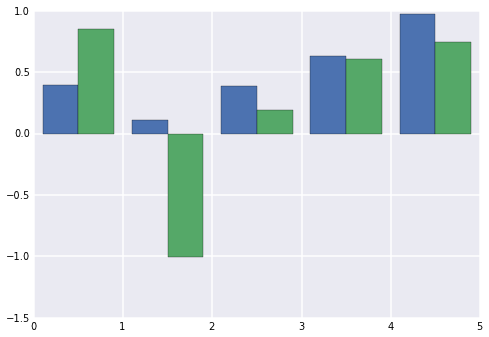We can quantify the reliability of our estimated weights with bootstrapping.

In :
iterations = 1000
w_boot = zeros((iterations, len(w_ols)))
for i in xrange(iterations):

# Get an index vector to sample with replacement
samp = randint(0, n_obs, n_obs)

# Resample data and model
samp_X = X[samp, :]
samp_y = y[samp]

# Fit the model on this iteration
w_boot[i, :] = ols_fit(samp_X, samp_y)

# Get the 95% confidence interval for each weight (across each column in the design matrix)
w_ols_ci = moss.percentiles(w_boot, [2.5, 97.5], axis=0)

In :
# The bar() function expects errorbar coordinates to be relative to bar height
# We have a convenience function in our plotting library to convert these
ebar_coords = seaborn.ci_to_errsize(w_ols_ci, w_ols)

# Plot the confidence intervals as error bars on our barplot from above
bar(arange(5) + .1, w, .4, label="actual weights")
bar(arange(5) + .5, w_ols, .4, yerr=ebar_coords, color=colors, ecolor="gray", label="estimated weights");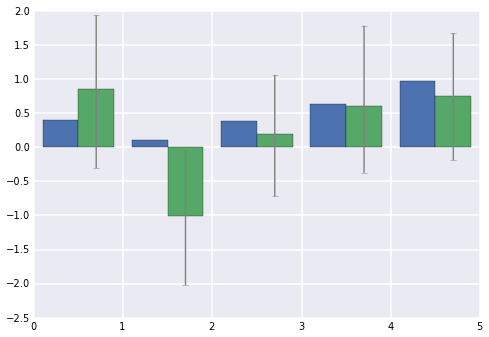### Reliability and Noise¶

Notice that the reliability of the estimates increases when we collect more data or have less noise in the data (higher signal to noise ratio).

In :
X = column_stack((randn(1000, 4), ones(1000)))
w = rand(5)

# Large sample high noise; N = 1000, noise std = 5
y_large_n = dot(X, w) + randn(1000) * 5

# Small sample low noise; high signal to noise ratio
y_lownoise = dot(X[:100], w) + randn(100)

# Small sample, high noise
y_noisy = dot(X[:100], w) + randn(100) * 5

# Bootstrap all three models
n_boot = 1000
w_boot1 = moss.bootstrap(X, y_large_n, n_boot=n_boot, func=ols_fit)
w_boot2 = moss.bootstrap(X[:100], y_lownoise, n_boot=n_boot, func=ols_fit)
w_boot3 = moss.bootstrap(X[:100], y_noisy, n_boot=n_boot, func=ols_fit)

# The median of these bootstrapped estimates are the weights for each model.
# You could also use the mean if you prefer.
w_model1 = median(w_boot1, axis=0)
w_model2 = median(w_boot2, axis=0)
w_model3 = median(w_boot3, axis=0)

# Compute the 95% confidence intervals on parameter estimates
pcts = [2.5, 97.5]
ci1 = moss.percentiles(w_boot1, pcts, axis=0)
ci2 = moss.percentiles(w_boot2, pcts, axis=0)
ci3 = moss.percentiles(w_boot3, pcts, axis=0)


Plot both estimates against the actual weights. Notice the errorbars are much smaller when there was less noise or more data. The intuition is that increasing the sample size or making higher signal to noise measurements will decrease the error on our parameter estimates

In :
barx = linspace(0, 1, 6)[:-1]
models = [w, w_model1, w_model2, w_model3]
cis = [None] + map(seaborn.ci_to_errsize, [ci1, ci2, ci3], models[1:])
for i, model in enumerate(models):
bar(barx + i, model, 0.2, yerr=cis[i], color=colors[i], ecolor="gray")
xticks([.5, 1.5, 2.5, 3.5], ["model", "large N", "low noise", "noisy"]);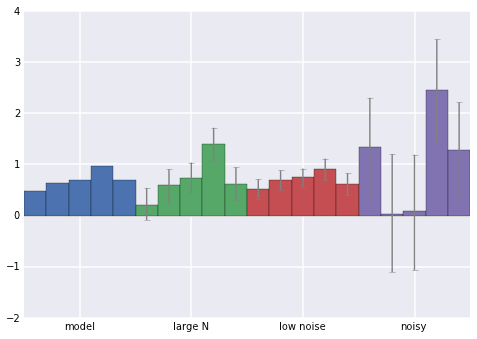### Model accuracy and noise¶

While the reliability of a parameter estimate depends both on the number of measurements that are made and the signal to noise of these measurements, the same is not true about model accuracy. We will explore this below.

In :
# We will use the R^2 function from the Scikit-learn function
from sklearn.metrics import r2_score
r2_model1 = r2_score(y_large_n, dot(X, w_model1))
r2_model2 = r2_score(y_lownoise, dot(X[:100], w_model2))
r2_model3 = r2_score(y_noisy, dot(X[:100], w_model3))

print 'Model 1 is reliable but not accurate. R^2 = %.2f' % r2_model1
print 'Model 2 is reliable and accurate. R^2 = %.2f' % r2_model2
print 'Model 3 is neither reliable nor accurate. R^2 = %.2f' % r2_model3

Model 1 is reliable but not accurate. R^2 = 0.10
Model 2 is reliable and accurate. R^2 = 0.74
Model 3 is neither reliable nor accurate. R^2 = 0.22


### Model reliability with correlated regressors¶

Now lets use bootstrapping to examine the effects of having correlated regressors. When each column (regressor) in the matrix is orthogonal the parameter estimates are much more reliable. High correlations between the columns means that decreasing the weight on one column can be compensated for by increasing the weight on another column. The result is that each estimated weight is very noisy and unreliable.

In :
# Here are the weights
w = rand(3)

# Simulate data from 3 uncorrelated regressors
X_orth = randn(100, 3)

# Signal with no noise
y_orth = dot(X_orth, w)

# Add noise that with a std proportional to the signal std
y_orth = y_orth + std(y_orth) * randn(100)

# Simulate data from 3 correlated regressors.  To do this first we define a covariance matrix, S.
cor = 0.8  # This is the correlation to induce between the variables
S = eye(3)
S[S == 0] = cor

# Then simulate data that comes from a 3-D gaussian (mean 0) with this covariance matrix
X_corr = random.multivariate_normal(zeros(3), S, 100)
y_corr = dot(X_corr, w)
y_corr = y_corr + std(y_corr) * randn(100)

# Bootstrap the model fits for the correlated and uncorrelated data
n_boot = 1000
boot_orth = moss.bootstrap(X_orth, y_orth, n_boot=n_boot, func=ols_fit)
boot_corr = moss.bootstrap(X_corr, y_corr, n_boot=n_boot, func=ols_fit)

# Calculate the 95% confidence intervals on the 2 sets of weights
pcts = [2.5, 97.5]
ci1 = moss.percentiles(boot_orth, pcts, axis=0)
ci2 = moss.percentiles(boot_corr, pcts, axis=0)


Plot the parameter estimates and 95% confidence intervals for the correlated and uncorrelated data. Notice how large the error bars are when the regressors are correlated. With correlated regressors you would need to collect much more data to reach an equivalent level of reliability.

In :
barx = linspace(0, 1, 4)[:-1]
models = [w, median(boot_orth, axis=0), median(boot_corr, axis=0)]
cis = [None] + map(seaborn.ci_to_errsize, [ci1, ci2], models[1:])
for i, model in enumerate(models):
bar(barx + i, model, 1. / 3, yerr=cis[i], color=colors[i], ecolor="gray")
xticks([.5, 1.5, 2.5], ["model", "orthogonal", "correlated"]);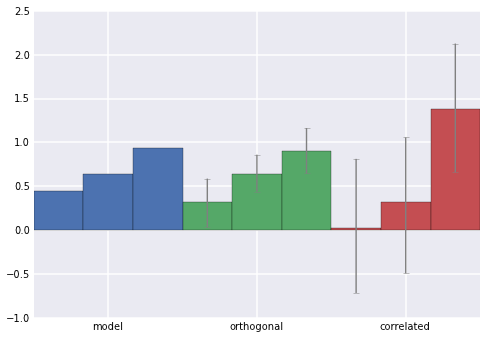### Reliability of complex models¶

The above scenarios of model reiability are slightly contrived. In the real world we rarely know what parameters to include in a model. Assessing reliability and accuracy of models is essential for determining the most apropriate set of parameters to include in a model.

In :
# Assume that this is the population of data
x_pop = randn(1000) + 4
noise = randn(1000) * 5
y_pop = 1.5 * x_pop ** 2 + noise

# And this is a random sample we collect in an experiment
n = 10
samp = permutation(arange(len(y_pop)))[:n]
x_samp = x_pop[samp]
y_samp = y_pop[samp]


When we plot the population and the sample data it is obvious that y is increasing as a funciton of X however with a small sample we can not reliably estimate the exponent on x.

In :
plot(x_pop, y_pop, "o", label="population")
plot(x_samp, y_samp, "o", color=colors, label="sample");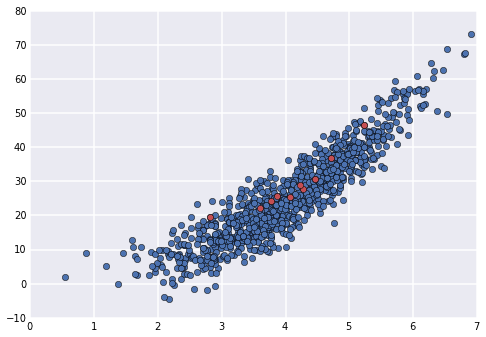We will bootstrap the parameter estimates for two models. Model 1 is a linear model and model 2 is a non-linear model with an exponent as a free parameter.

In :
from scipy.optimize import curve_fit
modelfunc = lambda x, a, b, c: a * x ** b + c
# Bootstrap the linear and nonlinear fitting procedures
w_ols_boot, w_nonlin_boot = [], []
for i in xrange(100):

# Get the bootstrap index
boot_samp = random.randint(0, n, n)
boot_x = x_samp[boot_samp]
boot_y = y_samp[boot_samp]

# Fit a linear model
w_nonlin_boot.append(curve_fit(modelfunc, boot_x, boot_y, maxfev=100000))
w_ols_boot = array(w_ols_boot)
w_nonlin_boot = array(w_nonlin_boot)


The parameter estimates for the non linear model are very noisy. Because there is so little data to constrain estimates, they very substantially from one bootstrap iteration to the next. For the linear model on the other hand there are fewer parameters and they are more reliable.

In :
# Visualize the reliability of the parameter estimates of the linear model
ols_model = median(w_ols_boot, axis=0)
pcts = [16, 84]
ols_ci = percentile(w_ols_boot, pcts, axis=0)
nonlin_model = median(w_nonlin_boot, axis=0)
nonlin_ci = percentile(w_nonlin_boot, pcts, axis=0)
ols_ebars = seaborn.ci_to_errsize(ols_ci, ols_model)
bar(range(2), ols_model, 1, yerr=ols_ebars, ecolor="gray", label="OLS")
nonlin_ebars = seaborn.ci_to_errsize(nonlin_ci, nonlin_model)
bar(range(2, 5), nonlin_model, 1, yerr=nonlin_ebars, ecolor="gray", color=colors, label="nonlinear")
xticks(arange(5) + .5, ["slope", "constant", "slope", "exponent", "constant"])
legend();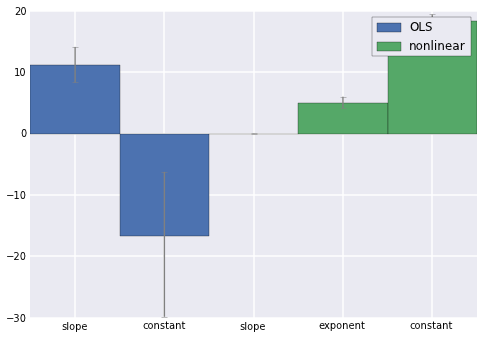### Visualize model fits¶

The code below provides an example of how to visualize a model's fit to the data including error bars on the model estimates. This code can be generalized to any complex model.

In :
scatter(x_pop, y_pop, facecolor='none')
l, r = xlim()
xx = linspace(l, r, 200)
# Loop over each bootstrap iteration and compute that models prediction for all of the x values
y_model = []
for i, w in enumerate(w_ols_boot):
y_i = dot(xx, w_ols_boot[i]) + w_ols_boot[i]
y_model.append(y_i)

# Plot the linear prediction and transparent error bars
pcts = [16, 84]
plot(xx, median(y_model, axis=0))
lin_ci = moss.percentiles(y_model, pcts, axis=0)
fill_between(xx, *lin_ci, color=colors, alpha=.2)

# Now derive the nonlinear predictions and plot
y_model_nlin = []
for i, w in enumerate(w_nonlin_boot):
y_i = modelfunc(xx, *w)
y_model_nlin.append(y_i)
plot(xx, median(y_model_nlin, axis=0))
nlin_ci = moss.percentiles(y_model_nlin, pcts, axis=0)
fill_between(xx, *nlin_ci, color=colors, alpha=.2);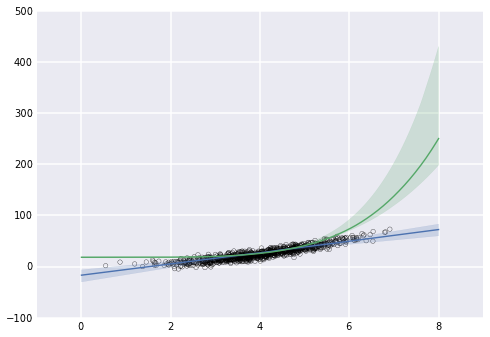Also note the distribution of parameter estimates for the non linear model. Extreme outliers are relatively common on the exponent. Notice that in the code above we plotted the median fit rather than the mean fit. Sometimes the mean can be outside the error bars! This is an instance when the mean is a poor estimate of the central tendency.

In :
scatter(x_pop, y_pop, facecolor='none')
plot(xx, mean(y_model_nlin, 0), label="mean", color=colors)
plot(xx, median(y_model_nlin, 0), label="median", color=colors)
legend(loc="best");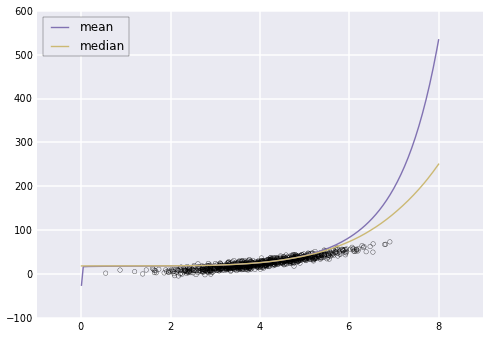Here is the histogram of parameter estimates for the non linear model. Note that the distribution of parameter estimates across bootstrap iterations is highly non-gaussian.

In :
figure(figsize=(9, 9))
plot_type = ["slope", "exponent", "constant"]
for pi in range(3):
subplot(3, 1, pi + 1)
hist(w_nonlin_boot[:, pi], 25, color=colors[pi])
title(plot_type[pi]);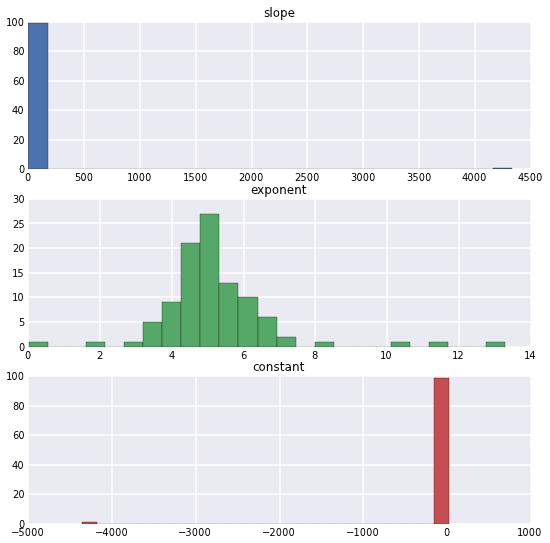Here is the histogram of parameter estimates for the linear model. Note that the distribution of parameter estimates across bootstrap iterations is roughly gaussian.

In :
figure(figsize=(9, 6))
plot_type = ["slope", "constant"]
for pi in range(2):
subplot(2, 1, pi + 1)
hist(w_ols_boot[:, pi], 25, color=colors[pi])
title(plot_type[pi]);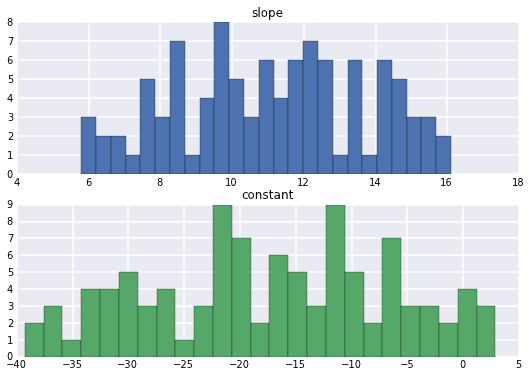Which model is more accuracte? This is a question for cross validation.